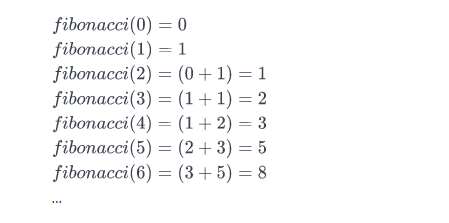# Find the Nth Fibonacci Number – C# Code

The Fibonacci sequence begins with Fibonacci(0) = 0  and Fibonacci(1)=1  as its respective first and second terms. After these first two elements, each subsequent element is equal to the sum of the previous two elements.

Here is the basic information you need to calculate Fibonacci(n):

• Fibonacci(0) = 0
• Fibonacci(1) = 1
• Fibonacci(n) = Fibonacci(n-1) + Fibonacci(n-2)

Given n, complete the fibonacci function so it returns Fibonacci(n).

Input Format

Nth number

Output Format

Fibonacci() Nth number.

Sample Input

Sample Output

Explanation

Consider the Fibonacci sequence:We want to know the value of Fibonacci(3). If we look at the sequence above,Fibonacci(3)  evaluates to 2. Thus, we print 2 as our answer.

This site uses Akismet to reduce spam. Learn how your comment data is processed.